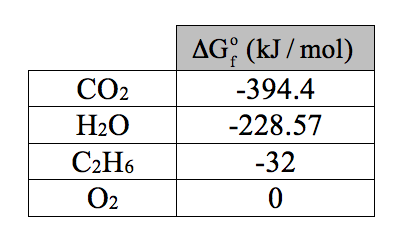# Problem: Use the data from this table of thermodynamic properties to calculate the maximum amount of work that can be obtained from the combustion of 1.00 mole of ethane, CH3CH3(g) at 25 °C and standard conditions.

###### FREE Expert Solution
83% (426 ratings)
###### FREE Expert Solution

We can calculate the maximum amount of work with the standard Gibbs free energy of the reaction using the relation below.

83% (426 ratings)###### Problem Details

Use the data from this table of thermodynamic properties to calculate the maximum amount of work that can be obtained from the combustion of 1.00 mole of ethane, CH3CH3(g) at 25 °C and standard conditions.What scientific concept do you need to know in order to solve this problem?

Our tutors have indicated that to solve this problem you will need to apply the Gibbs Free Energy concept. You can view video lessons to learn Gibbs Free Energy. Or if you need more Gibbs Free Energy practice, you can also practice Gibbs Free Energy practice problems.

What is the difficulty of this problem?

Our tutors rated the difficulty ofUse the data from this table of thermodynamic properties to ...as medium difficulty.

How long does this problem take to solve?

Our expert Chemistry tutor, Sabrina took 6 minutes and 18 seconds to solve this problem. You can follow their steps in the video explanation above.

What professor is this problem relevant for?

Based on our data, we think this problem is relevant for Professor Blake's class at UCI.